# High School Math : Simplifying Logarithms

## Example Questions

### Example Question #4 : Exponential And Logarithmic Functions

Solve for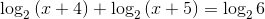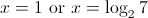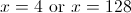The correct solution set is not included among the other choices.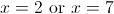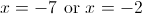The correct solution set is not included among the other choices.

Explanation: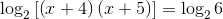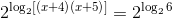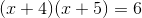FOIL: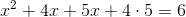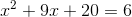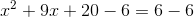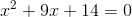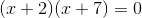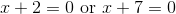These are our possible solutions. However, we need to test them.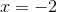: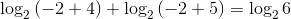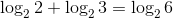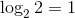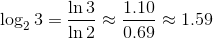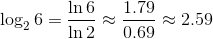The equation becomes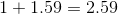. This is true, sois a solution.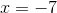: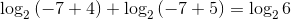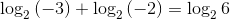However, negative numbers do not have logarithms, so this equation is meaningless.is not a solution, andis the one and only solution. Since this is not one of our choices, the correct response is "The correct solution set is not included among the other choices."